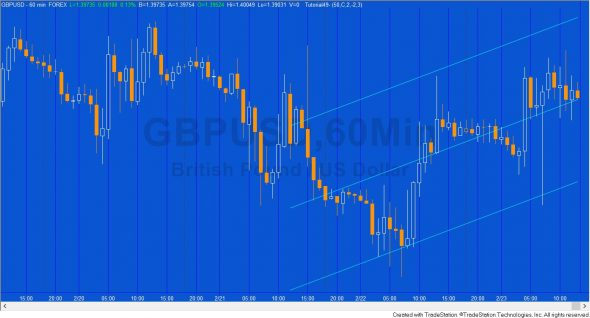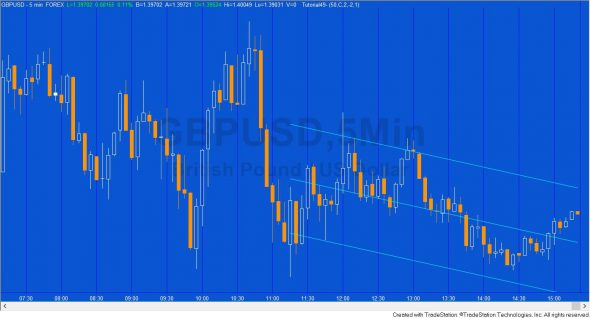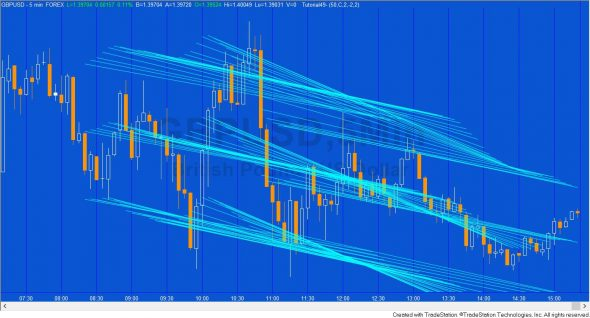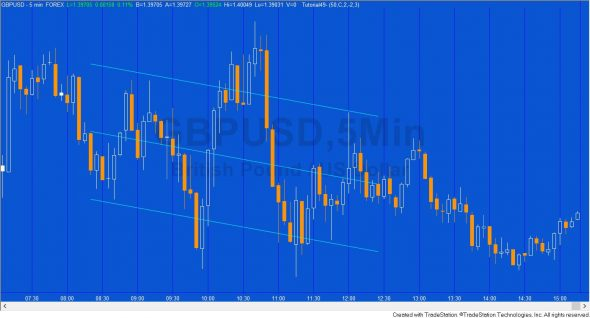# Tutorial 49 | Creating a linear regression channel

Note: Tutorial 49 has been updated to include the original tutorial program together with an updated version that allows you to modify the style, color and thickness of the lines. The two programs are included in one ELD file. See purchase instructions below.

Both tutorial programs work with TradeStation (8.7 forward) and MultiCharts.

This tutorial uses the code originally created in tutorial 48 that created a line using linear regression that best fits chart data. The linear regression technique used in this tutorial uses the least squares method to calculate the slope and y-intercept of this line.

The standard deviation of the right most end of the regression line is calculated in order to create a channel made up of two parallel lines above and below the linear regression line. These lines are drawn a user input multiple of standard deviations above and below the original linear regression line.

Finally the tutorial explains how to modify the program developed in tutorial 48 to calculate the standard deviation of the linear regression line’s end point.

Tutorial 49 was created using TradeStation 8.7 and works with all later versions of TradeStation and with Multicharts.Tutorial 49 applied to a 60 minute GBPUSD chart. A linear regression line with parallel channels drawn 2 standard deviations above and below the line.

The tutorial includes three different options for displaying the channel:

### Option 1

1. In the first option the channel is always moved to the end of the chart to apply to the last NumBars bars.### Option 2

2. A new channel is drawn every time a new bar is printed.### Option 3

3. The channel is drawn when the show me study is first applied to the chart and doesn’t move.You might also be interested in program 63. Program 63 draws linear regression lines, and then creates a smoothed plot based on the linear regression end point. It also offers the option to plot oscillators based on non-smoothed plots.